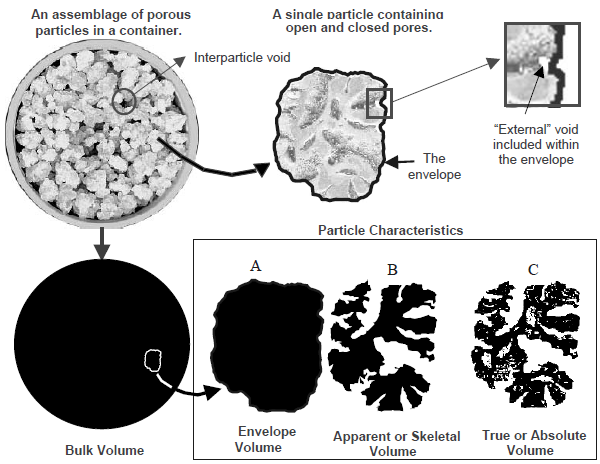Categories

## What is density? how to distinguish different density definitions?

As material researchers, we know density is the physical property of materials. Even since grade school, we are taught that density is simply the mass of an object divided by its volume.

However, this is pretty complicated in the case of cementitious materials. The mass of a certain amount of harden cement (or concrete) paste is a finite value, but how about the volume? since the harden cement paste is porous, how do we consider the open and close pores inside the paste as we want to determine the volume?

I met the problem when I performed Mercury Intrusion Porosimetry (MIP) test, especially when I read the output result from the MIP machine, there are bulk density, skeletal density, envelope density and apparent density.

It is not difficult to find these definitions from either textbooks or internet. First I list them below:

Apparent particle density: The mass of a particle divided by its apparent (particle) volume (BSI).

Bulk density: (also called Bulk powder density): The apparent powder density under defined conditions.

The mass of the particles divided by the volume they occupy that includes the space between the particles (ASTM D5004).

The ratio of the mass of a collection of discrete pieces of solid material to the sum of the volumes of: the solids in each piece, the voids within the pieces, and the voids among the pieces of the particular collection (ASTM D3766).

Envelope density: The ratio of the mass of a particle to the sum of the volumes of: the solid in each piece and the voids within each piece, that is, within close-fitting imaginary envelopes completely surrounding each piece (ASTM D3766). The ratio of the mass of a particle to the envelope volume of the particle (implied by BSI).

Skeletal density: The ratio of the mass of discrete pieces of solid material to the sum of the volumes of: the solid material in the pieces and closed (or blind) pores within the pieces (ASTM D3766).

True density (also called True particle density): The mass of a particle divided by its volume, excluding open pores and closed pores (BSI).

It is a headache to me to well understand them and distinguish these different density definitions. I guess it is still not clear for people to understand these densities either. Fortunately, I found a schematic picture (below) well illustrate the physical meaning of these density definitions, which you may no longer misunderstand them any more.Is it now clear?